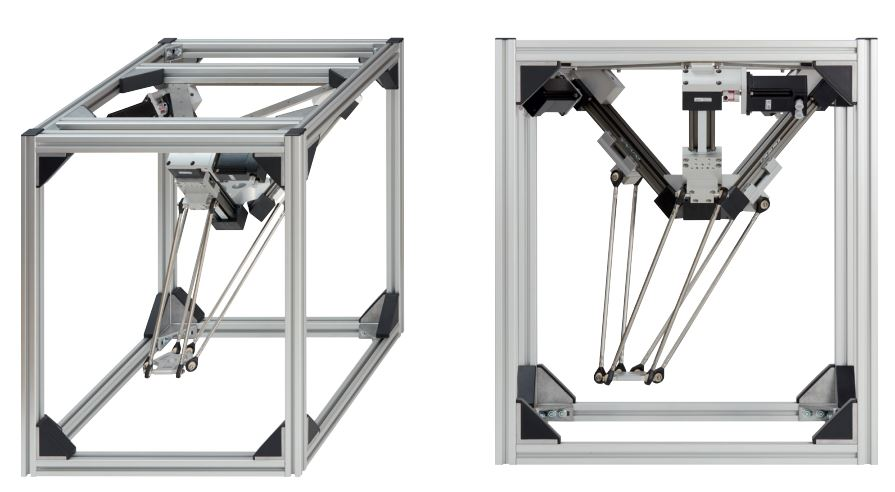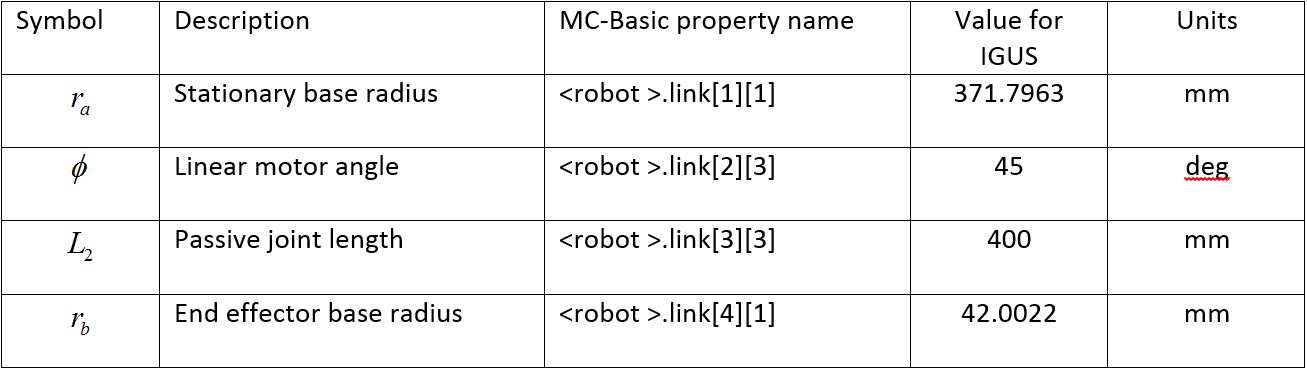DELTA robot - linear model

The general linear DELTA robot is usually from the following configuration:

The Delta Robot belongs to a group called parallel robots. Parallel robots use multiple links attached to the end effector in order to move. In most cases, the motors used to drive parallel robots are mounted to the stationary frame of the robot, and do not move.

The linear robot Delta is a 3 degrees of freedom well known parallel structure allowing the 3 natural translations X, Y and Z. It exists with either rotational or linear actuated joints

The basic idea behind the Delta parallel robot design is the use of parallelograms. A parallelogram allows an output link to remain at a fixed orientation with respect to an input link. The use of three such parallelograms restrains completely the orientation of the mobile platform which remains only with three purely translational degrees of freedom. The input links of the three parallelograms are mounted on rotating levers via revolute joints. The revolute joints of the rotating levers are actuated in two different ways: with rotational motors or with linear actuators. Finally, a fourth leg is used to transmit rotary motion from the base to an end-effector mounted on the mobile platform.

WIPO patent issued on June 18, 1987 (WO 87/03528):

The kinematics of the linear Delta allows a large travel space (as large as desired) in one direction (in the xdirection of the horizontal linear delta and in the zdirection for the vertical linear Delta). On the other hand, the dominant advantage of the linear Delta is its good sensitivity (and hence its resolution) in the direction3 of z. The actuator efforts are closest to the tool efforts, which makes it more suitable for tool machining applications and for applications needing stiffness. Linear sensors can be integrated to the linear guide ways and hence improve the precision of the structure.

The vertical type linear Delta is the most adapted to have the maximum stiffness in the vertical direction. Thanks to the ternary symmetry of the motor’s disposition, it is also the most suitable to have a convenient distribution of the sensitivity in the global workspace.

To improve the stiffness of the linear vertical Delta in the extremities, The following configuration has been applied. It is close to the rotational Delta but has one additional parameter, namely the angle (or the angles for non symmetrical versions) between the vertical and the robot axis linear guideways direction. The travel in the z direction has been reduced but we gain in the stability in the horizontal direction. The workspace of the robot is relatively large with respect to the overall robot size.DELTA Work-SpaceLinear Delta Workspace assessment

The linear Delta kinematics works with points of the XYZR robot type (X, Y , Z and ROLL) where the three coordinates are always expressed in millimeters.

The DELTA WORLD workspace is limited by joint limits and the following auxiliary cartesian boundaries, available for the user:

• RMAX – maximum radius of a cartesian point (${\sqrt {x^{2}+y^{2}+z^{2}}}$), no points beyond this radius are allowed.
• RMIN - smallest radius of a cartesian point (${\sqrt {x^{2}+y^{2}+z^{2}}}$). No points inside this radius are allowed.
• ZMIN – z coordinate of the “lowest” robot position. No points below this plane are allowed.

Robot configurations

Delta robot has no configuration flags, there is always one unique solution of the inverse kinematics problem. This further means that the configuration arguments in inverse kinematics function ToJoint have no influence on the result. Also Cartesian movements like MOVES, CIRCLE or PASS THROUGH are not influenced by any of the command configuration flags (acmd, ecmd, wcmd), the motion will be the same independent of the value of any of these flags.

Singular points

Delta robot, contrary to the open-kinematics chain robot has no singular joint positions where the joint velocities tend to infinity but there are so called “direct kinematics singularity” where the stiffness of the robot is significantly reduced.

Geometric parameters of the DELTA robot

For the linear DELTA shown above we have the following parameters:The above parameters of link matrix property of the robot must be filled-in in the robot’s setup file. In order that the system accepts the above setup the “configgroup” command must be executed. The model is assuming that the three base motors are rotated for exactly 120 degrees. Also it has been assumed that all three lags are of the same dimensions. The WORLD coordinate system is based in the center of the motor plane. Note that only the above set of parameters affects the robot geometry, the rest of the link[][] and axis[][] values do not influence the robot geometry in any way and are not affected (changed) by configgroup command or any other robot command.